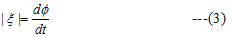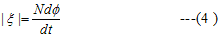## Faradays law of electromagnetic induction

• From experiments on EM induction, faraday came to conclude then an emf was being induced in the coil when the magnetic flux linked to it was being changed either by
(i) Moving magnet close or away from the coil(experiment 1)
(ii) Chnaging amouny of current in the primary coil or motion of either of the coils relative to each other (experiment 2)
• From these observations faraday enunciated an important law. The magitude of the induced emf produced in the coil is given bywhere Φ is the magnetic flux as given by equation (1) .ξ is expressed in volts
• If the circuit is a tightly wound coil of N turns then total flux linked by N turns coil is NΦ.So induced EMF is whole coil isAbove equation only give the magnitude of the induced EMF and it does not give its direction.
• we know that unit of magnetic flux is 1 weber=1 T.m2
• If magnetic flux linking a single turn changes at a rate of 1weber per second then Induced emf=Magnetic flux/time=1 weber/sec Now since weber=Nm/Amp
Hence 1 weber/sec= 1 N.m/A.s =1 Joule/coulumb
• Hence the unit of emf is volt.